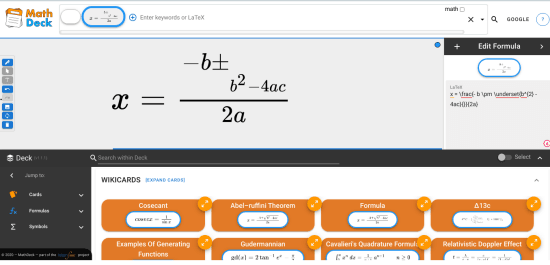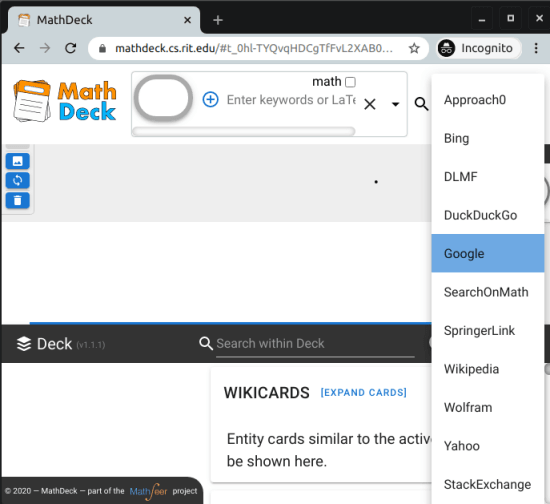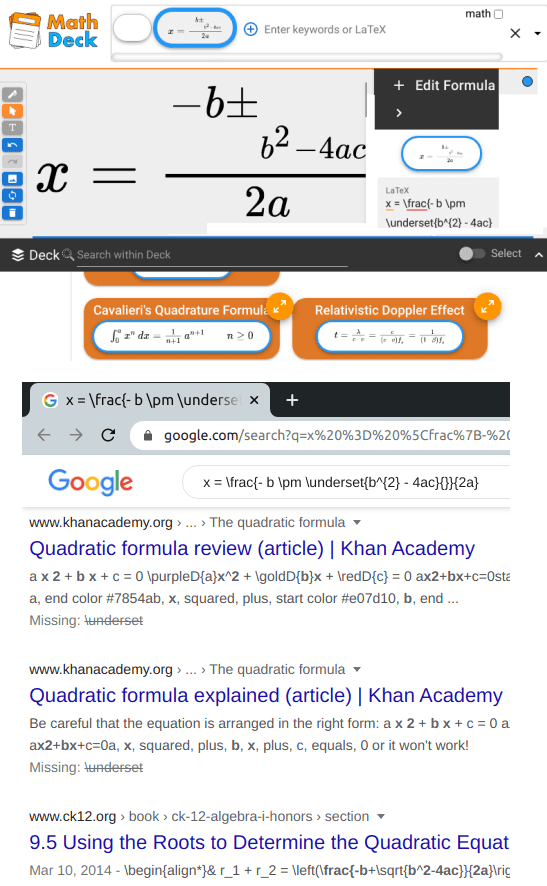Editor Ratings:
User Ratings:
[Total: 0 Average: 0]

MathDeck is a free website where you can search math equations using LaTeX. Here it lets you search for Math equation terms using various search engines and see the results. The best part is that here you can write math equation on a canvas as well in order to search them on the internet. It lets you search the equations on Google, Bing, Wolfram, DuckDuckGo, DLMF, Approach0, Springer, and some others. You can just write your equation there with free hand or use LaTeX to do that. Also, you can simply upload an image from your PC that has an equation and it will search that for you.

If you are into math then you often need to find some resources but you can’t type equations in search engine box. That is where MathDeck comes in. Here you can write anything in Math that you want to search. You can choose the list of various search engines that it supports and get the results. And if you don’t know LaTeX code of some equation then you can draw one on the canvas and let it generate that for you. And there is no need to register on it in order to use it.## Search Math Equations using LaTeX on Google, StackExchange: MathDeck

You can go to the main MathDeck website from here. The UI is very simple and you can just start using it right away as there is no sign up or registration is required. There is search bar on the top of the website and then default search engine is set to Google. There is a canvas on which you can draw an equation and it will change that to text for you by generating the LaTeX code.Now, to search some equation related to math, you need to just write an equation. Or, you can simply upload an image for your PC. Whatever equation you type, it will add it to the search box. And then you can just hit the search button and see the corresponding results. You can change the search engines if you want to see different results. For example, if you want to see academic results, then change it to Springer.This is how you can use simple and powerful Math search website. It has almost everything that you need. You just enter whatever equation you want to search for and get the corresponding results. It works with almost all type of Math equations and use its OCR capability to get equations from images as well.

### Closing thoughts

If you are looking for a powerful website to search math equations then you are at right place. Simply use MathDeck and get results from various search engines. I really liked the fact that here you can search math related stuff using LaTeX. Also, I really liked the UI of the tool and options to use different search engines to get different results.